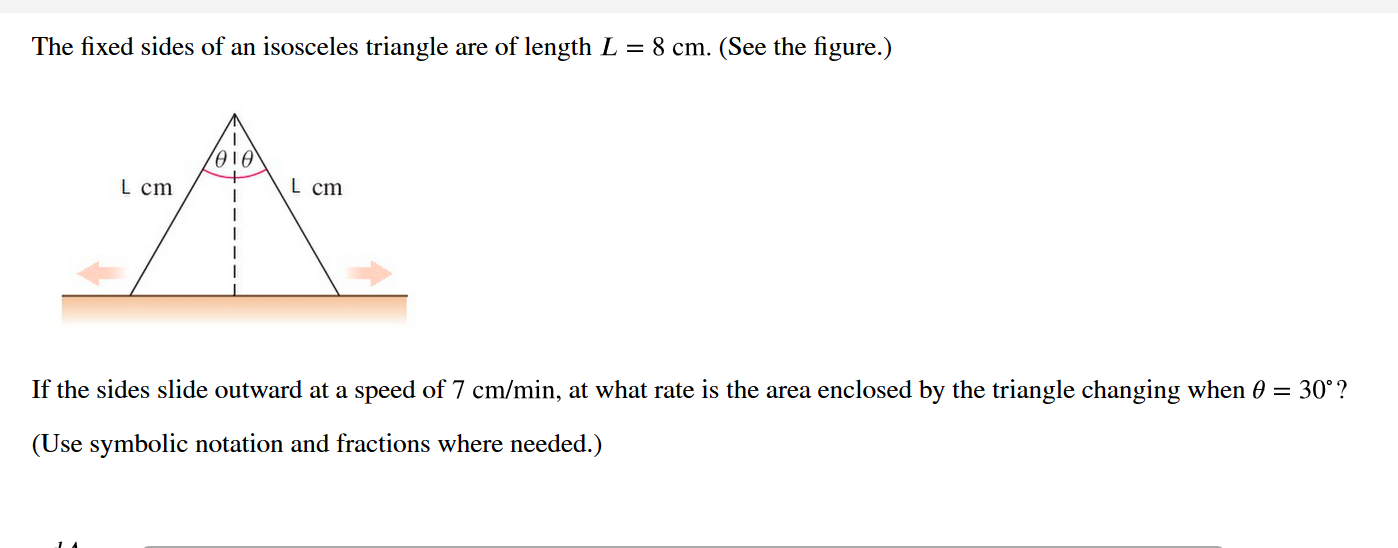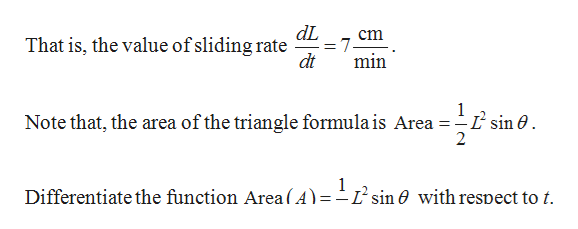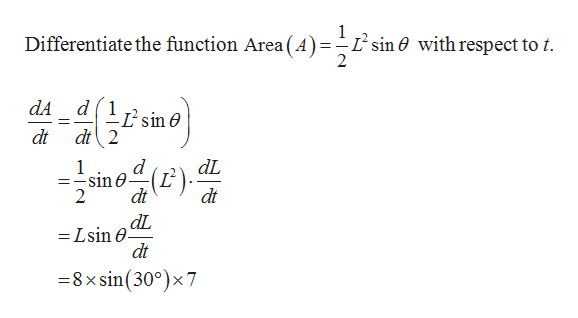# The fixed sides of an isosceles triangle are of length L = 8 cm. (See the figure.)L cmL cmIf the sides slide outward at a speed of 7 cm/min, at what rate is the area enclosed by the triangle changing when 0 = 30°?(Use symbolic notation and fractions where needed.)

Question
54 viewshelp_outlineImage TranscriptioncloseThe fixed sides of an isosceles triangle are of length L = 8 cm. (See the figure.) L cm L cm If the sides slide outward at a speed of 7 cm/min, at what rate is the area enclosed by the triangle changing when 0 = 30°? (Use symbolic notation and fractions where needed.) fullscreen
check_circle

Step 1

Given that, the length of the triangle is L = 8 cm and the sliding rate of the side is 7 centimeter per second.help_outlineImage TranscriptionclosedL cm That is, the value of sliding rate 7 min dt 1 L sin 0 2 Note that, the area of the triangle formula is Area Differentiate the function Area ( A)=-sin 0 with respect to t. fullscreen
Step 2help_outlineImage TranscriptioncloseDifferentiate the function Area (A)=-L'sin6 sin e with respect to t d1 Lsin e dA dt 2 dt d sine dt ainE) dL dt 2 dL = Lsin 0 dt =8 xsin(300)x 7 fullscreen

### Want to see the full answer?

See Solution

#### Want to see this answer and more?

Solutions are written by subject experts who are available 24/7. Questions are typically answered within 1 hour.*

See Solution
*Response times may vary by subject and question.
Tagged in

### Other